Hyperbolic functions

(diff) ← Older revision | Latest revision (diff) | Newer revision → (diff)

The functions given by the formulas: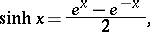(1)

the hyperbolic sine; and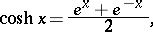(2)

the hyperbolic cosine. The hyperbolic tangentis also sometimes considered. Other notations include: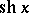,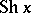,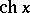,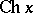,,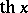,. The graphs of these functions are shown in Fig. a.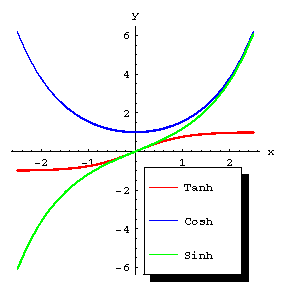Figure: h048250a

The principal relations are: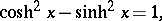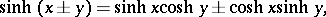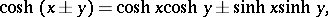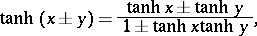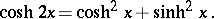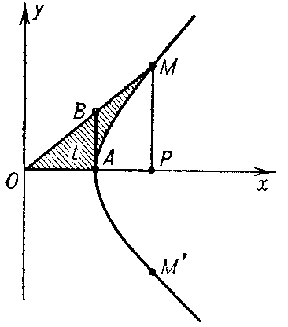Figure: h048250b

The geometrical interpretation of hyperbolic functions is similar to that of the trigonometric functions (Fig. b). The parametric equations of hyperbolas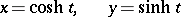make it possible to interpret the abscissa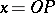and the ordinateof a pointon the equilateral hyperbolaas the hyperbolic sine and cosine; the hyperbolic tangent is the segment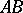. The parameterof the pointequals twice the area of the sector, whereis the arc of the hyperbola. The parameteris negative for a point(for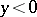).

The inverse hyperbolic functions are defined by the formulas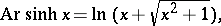The derivatives and basic integrals of the hyperbolic functions are: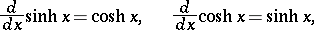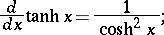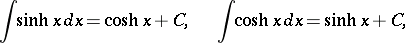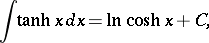The hyperbolic functionsandmay also be defined by the series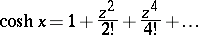in the entire complex-plane, so that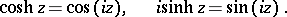(3)

Extensive tabulated values of hyperbolic functions are available. The values of the hyperbolic functions may also be obtained from tables giving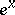and.